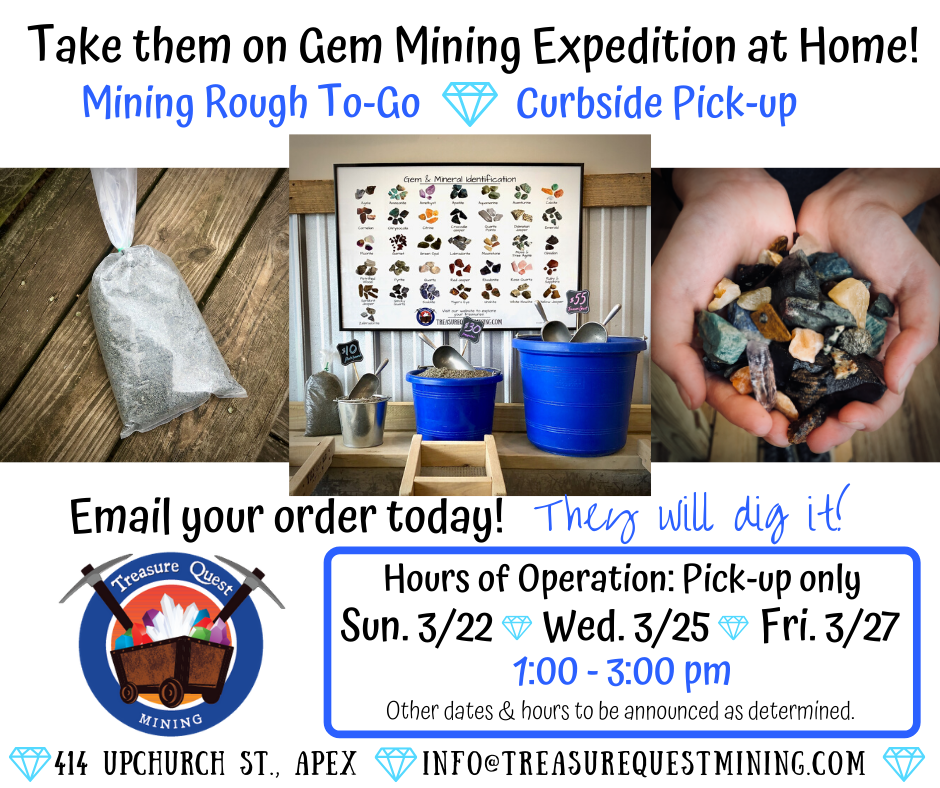# It can be tough being at home for an unknown period of time. We are excited to see so many businesses offering virtual classes! Here are some fun suggestions to break up your day!

 table div table+table+table div table{width:100%;padding:0}table div table+table+table div table img{width:96.23%;padding:0;float:none}table div table+table+table div table td{width:100%;padding:0 1.88% 18px}/* styles */table div table+table+table+table div table,table.module-3{width:37.74%;float:right;padding:0}table div table+table+table+table div table a{border:0 none;text-decoration:none}table div table+table+table+table div table img{width:100%!important;border:0 none;text-decoration:none}table div table+table+table+table div table td{width:100%;padding:0 0 20px 20px}/* styles */
 table div table+table+table+table+table div table{width:100%;padding:0}table div table+table+table+table+table div table img{width:96.23%;padding:0;float:none}table div table+table+table+table+table div table td{width:100%;padding:0 1.88% 18px}/* styles *//* styles */
 table div table+table+table+table+table+table+table div table{width:100%;padding:0}table div table+table+table+table+table+table+table div table img{width:96.23%;padding:0;float:none}table div table+table+table+table+table+table+table div table td{width:100%;padding:0 1.88% 18px}/* styles *//* styles */
 table div table+table+table+table+table+table+table+table+table div table{width:100%;padding:0}table div table+table+table+table+table+table+table+table+table div table img{width:96.23%;padding:0;float:none}table div table+table+table+table+table+table+table+table+table div table td{width:100%;padding:0 1.88% 18px}/* styles *//* styles */
 table div table+table+table+table+table+table+table+table+table+table+table div table{width:100%;padding:0}table div table+table+table+table+table+table+table+table+table+table+table div table img{width:96.23%;padding:0;float:none}table div table+table+table+table+table+table+table+table+table+table+table div table td{width:100%;padding:0 1.88% 18px}/* styles *//* styles */
 table div table+table+table+table+table+table+table+table+table+table+table+table+table div table{width:100%;padding:0}table div table+table+table+table+table+table+table+table+table+table+table+table+table div table img{width:96.23%;padding:0;float:none}table div table+table+table+table+table+table+table+table+table+table+table+table+table div table td{width:100%;padding:0 1.88% 18px}/* styles */# Treasure Quest Mining

Take the kids on a gem mining expedition at home! Treasure Quest Mining, an indoor gem mine and prehistoric gift shop in Apex, has to-go bags and family-sized buckets of mining rough available with curbside service. Wondering how to mine at home? It’s easier than you might think! Wash your mining rough in a sand sifter or pasta colander by dunking it in a water basin or rinsing it with a hose to reveal your treasure. Pick-up days are adjusted with demand and are posted on their website, presently the store offers pick-up hours on Sunday, Wednesday and Fridays from 1:00 - 3:00. Email info@treasurequestmining.com to place your order.

# ~ Fun4RaleighKids Team

 table div table+table+table+table+table+table+table+table+table+table+table+table+table+table+table+table+table div table{width:100%;padding:0}table div table+table+table+table+table+table+table+table+table+table+table+table+table+table+table+table+table div table table{padding:0;float:left!important;width:52.755%!important}table div table+table+table+table+table+table+table+table+table+table+table+table+table+table+table+table+table div table td{padding-left:74px;padding-right:74px}table div table+table+table+table+table+table+table+table+table+table+table+table+table+table+table+table+table div table table td,table div table+table+table+table+table+table+table+table+table+table+table+table+table+table+table+table+table div table table+table td{padding-left:0;padding-right:20px}table div table+table+table+table+table+table+table+table+table+table+table+table+table+table+table+table+table div table table+table{float:left!important;width:26.246%!important}table div table+table+table+table+table+table+table+table+table+table+table+table+table+table+table+table+table div table table+table+table{float:left!important;width:20.998999999999995%!important}table div table+table+table+table+table+table+table+table+table+table+table+table+table+table+table+table+table div table table+table+table td{padding-left:0;padding-right:0}/* styles */